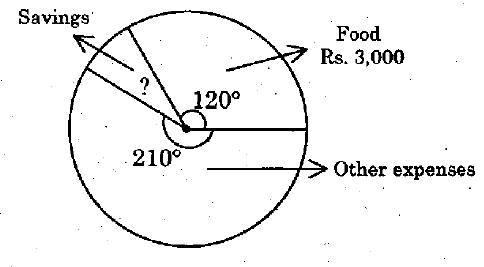# Group 4 2014 December GE TNPSC Question Paper

## Group 4 2014 December GE TNPSC Questions

21.
Divide Rs. 700 among A, B, C so that A gets 2 times more than to B and B gets 2 times to C how much of amount they are getting?
A.
Rs. 100, Rs. 200, Rs. 400
B.
Rs. 200, Rs. 300, Rs. 200
C.
Rs. 300, Rs. 200, Rs. 200
D.
Rs. 400, Rs. 200, Rs. 100
D. Rs. 400, Rs. 200, Rs. 100
22.
A cone, hemisphere, and cylinder have equal bases. If the heights of the cone and a cylinder are equal and are same as the common radius then find the ratio of their respective volumes
A.
2 : 3 : 4
B.
1 : 2 : 3
C.
2 : 1 : 3
D.
3 : 2 : 5
B. 1 : 2 : 3
23.

Using Identities x - y = -6 ; xy = 4. Find the value of x3 - y3

A.

-288

B.

-388

C.

-268

D.

-278

A. -288

24.

Two taps can fill a tank in 30 minutes and 40 minutes. Another tap can empty it in 24 min. If the tank is empty and all the three taps are kept open, in how much time the tank will be filled

A.

1 1/2 hours

B.

two hours

C.

one hour

D.

2  1/2 hours

C. one hour

25.
The radius of a wheel is 1.75. How many revolutions will it make in travelling 11 km?
A.
10
B.
100
C.
1000
D.
10000
C. 1000
26.

Monthly expenditure of a person is shown in the figure. Find the amount has in his savings?A.

Rs. 800

B.

Rs. 900

C.

Rs. 1,000

D.

Rs. 750

D. Rs. 750

27.
When was the uniform pattern of education policy introduced in our country?
A.
1965
B.
1968
C.
1970
D.
1971
B. 1968
28.
FERA is _________ Exchange Regulation Act.
A.
Foreign
B.
Forest
C.
Fiscal
D.
Finance
A. Foreign
29.
The first five year plan of India was launched in
A.
1947
B.
1951
C.
1956
D.
1961
B. 1951
30.

I. Nimbus clouds are vertical clouds

II. Nimbus clouds are high clouds

III. Nimbus clouds are low clouds

IV. Nimbus clouds are cotton-like clouds

A.

I only

B.

II only

C.

III only

D.

III and IV